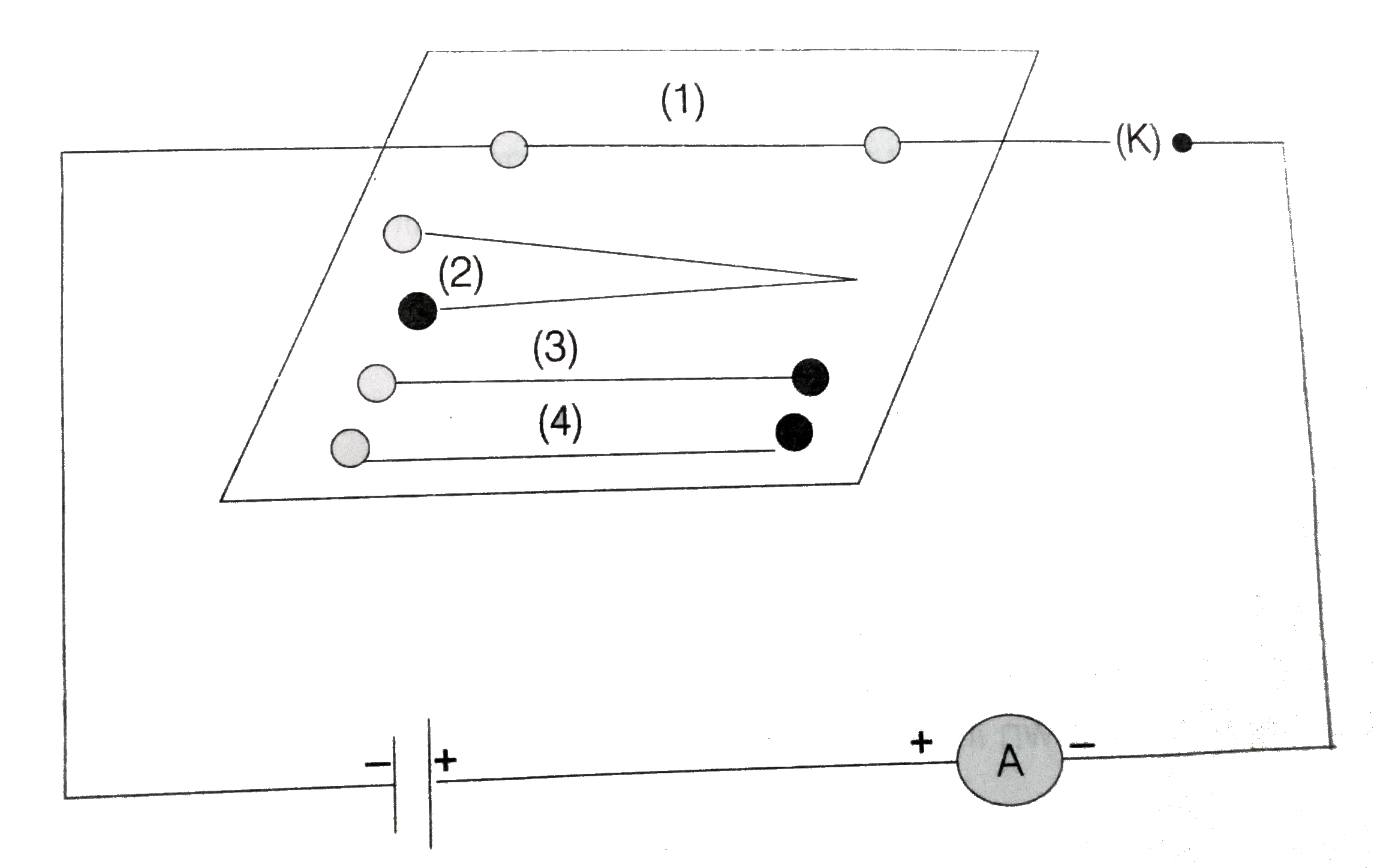# What is electrical resistivity ? How does it depend om temperature. Give its SI unit.

76 views
in Physics
closed
What is electrical resistivity ? How does it depend om temperature. Give its SI unit.

by (71.7k points)
selected by

The electrical resistivity of a material is defined as the resistance of a conductor made of that material of unit length and unit cross-sectional area. Its SI unit is Ohm metre (Omegam).
R = p(l)/(A) rArr p = (RA)/(l) " "({:("where,", A = 2m^(2)),(,l = 1m):})
Consider an electric circuit consisting of a cell, an ammeter, a nichrome wire of length L (marked 1 to 4) and a plug key as shown belowAt point plug the key and note the current in ammeter. Replace the nichrome wire by another nichrome wire of same thickness but twice the length that is 2l at point 2. Again note the reading. Now, replace the wire by a thicker nichrome wire of same length / (marked 3). A thicker wire has a larger cross-sectional area.
Again note down the current through the circuit. Replace nichrome wire with copper wire of same length and same area of cross-section at point 4. Note the value of current. Notice the difference in the current in all cases. When the length of the wire is doubled, then the ammeter reading decreases to half its previous value i.e., current through the wire is halved. Since, resistance of the wire R = (V)/(l), then R is doubled which implies R prop L
When the nichrome wire is replaced by a thicker one of same material and length, the current in the wire increases which means that the resistance of the thicker wire (3) is less than that of the thinner wire (1). This implies R prop (1)/(A).
When the nichrome wire is replaced by a copper wire (4) of the same length and same cross-sectional area, then the current recorded by the ammeter is more. This means that the resistance of copper wire is less than that of the nichrome wire of tha same dimensions i.e., the resistance of the wire depends on the nature of its material.